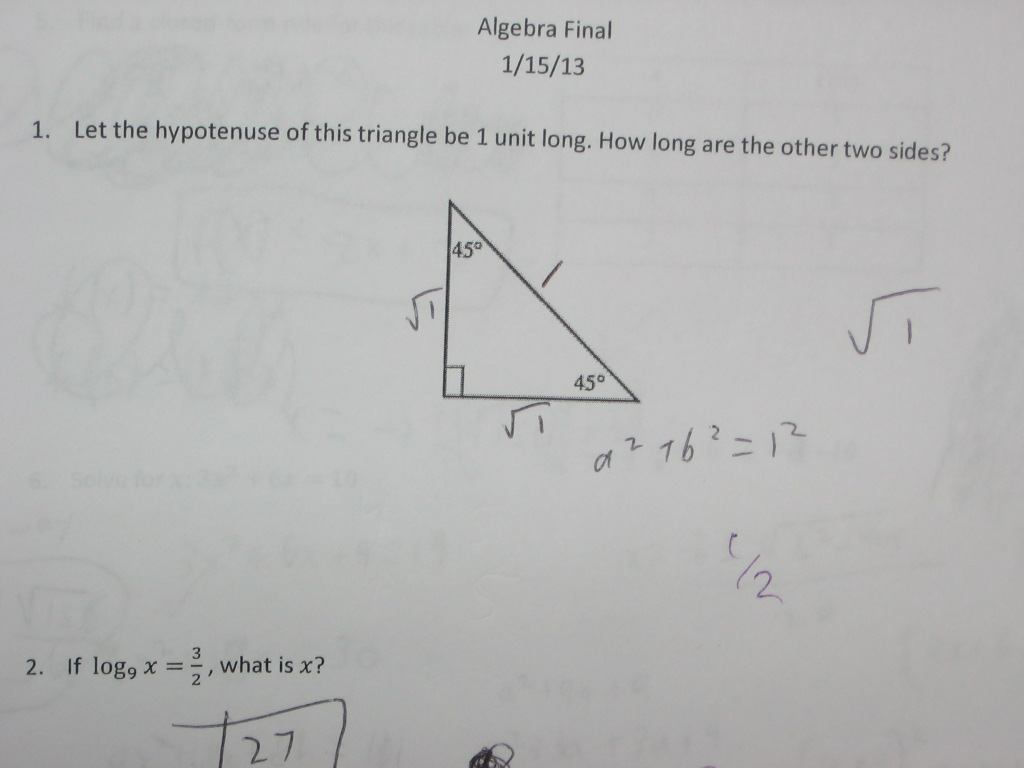Categories

# Sqrt(1) and Right TrianglesThis mistake seems ripe for theorizing. Would a kid make this mistake if the hypotenuse was 4 instead of 10?

## 4 replies on “Sqrt(1) and Right Triangles”

Hard to say exactly what their mistake was…if they thought 1 squared=2, then they are on track for an answer of the square root of one. The 1/2 below could mean they are taking half of the 1 squared, or they think that the square root of 1 is 1 is 1/2. This is definitely one where i’d have to look elsewhere in the test to see if this student is consistently misinterpreting square roots, or if it is a special case because of the 1.

When I look at this, it looks like a rushing mistake. I note that they didn’t show their work other than the a^2 + b^2 = 1^2. They knew that the 45 degree angles implied that a = b, but didn’t go step by step in their work and tried to skip a step. I’d think that the fact that they left the answer as sqrt(1) instead of simplifying that is also a characteristic of rushing.

I agree with afterMath’s first couple of sentences, but disagree with the last one — I know plenty of kids who have trouble simplifying sqrt(0) and sqrt(1) when they have no trouble with sqrt(4) or sqrt(9).

khanhdangsays:

I agree with the comment by afterMath because they say it’s a rushing mistake. I think this could be it because it showed that they knew what the Pythagorean formula was, but even then they didn’t plug in their answers to the formula to recheck it.